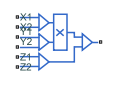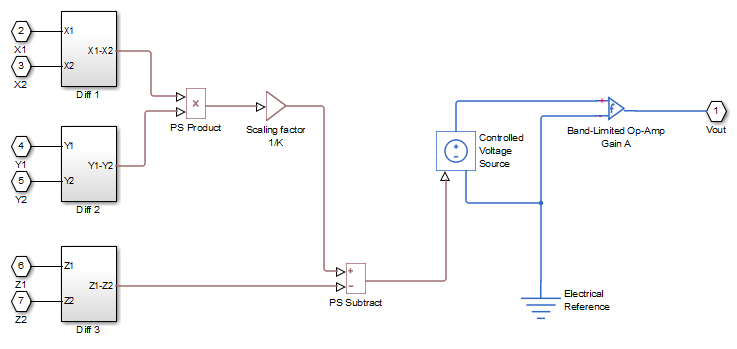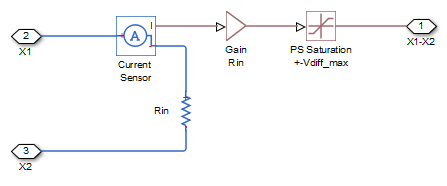# Multiplier

Integrated circuit multiplier

• Library:
• Simscape / Electrical / Integrated Circuits

•## Description

The Multiplier block models an integrated circuit multiplier. The block implements the following equation, which defines the voltage applied to the output port:

`${V}_{out}=A\left(\frac{\left({X}_{1}-{X}_{2}\right)\left({Y}_{1}-{Y}_{2}\right)}{K}-\left({Z}_{1}-{Z}_{2}\right)\right)$`

where X1, X2, Y1, Y2, Z1, Z2 are the voltages presented at the input ports, A is the gain, and K is the scale factor.

In a typical multiplication circuit, the output is fed back into input Z1, which results in the following gain (assuming that A is large):

`${V}_{out}=\left(\frac{\left({X}_{1}-{X}_{2}\right)\left({Y}_{1}-{Y}_{2}\right)}{K}+{Z}_{2}\right)$`

The value of the scale factor K is usually altered by an external resistor bias network. The Multiplier block implements K as an internal gain, and the external bias network is not necessary for system simulation. A typical value for K is 10, with a typical adjustment down to 3.

You can use the Multiplier block to implement a number of other functions, as well as multiplication. Examples include division, squares, and square roots. For example circuits, consult manufacturer datasheets.

The following figure shows the internal model structure of the Multiplier block. It includes the Band-Limited Op-Amp block to model finite bandwidth and slew-rate limiting.The next figure shows one of the differential subsystem blocks. All three differential subsystem blocks are identical in structure.## Assumptions and Limitations

• Only differential limiting of the inputs is implemented. You must ensure that the absolute values of the inputs you use keep the actual device operating in its linear region.

• Output current is such that the integrated circuit is operating in the linear I-V region, which can be approximated by a voltage source plus a series output resistance.

• Input offset voltage is not modeled, and the input voltage-current relationship is treated as linear within the differential signal voltage range.

## Ports

### Conserving

expand all

Electrical conserving port associated with the multiplier X1 non-inverting input.

Electrical conserving port associated with the multiplier X2 inverting input.

Electrical conserving port associated with the multiplier Y1 non-inverting input.

Electrical conserving port associated with the multiplier Y2 inverting input.

Electrical conserving port associated with the multiplier Z1 non-inverting input.

Electrical conserving port associated with the multiplier Z2 inverting input.

Electrical conserving port associated with the multiplier output port.

## Parameters

expand all

### Main

The scaling factor K in the equation that defines output voltage. Datasheets sometimes refer to it as the scale factor, or SF.

The gain of the internal operational amplifier, corresponding to the gain A in the equation that defines output voltage.

### Inputs

Each of the differential inputs is approximated as a linear resistor with value Rin. Set this value to the datasheet value for differential resistance.

This value, Vdiff_max, is used to limit the magnitude of each of the three differential input voltages. Set this value to the datasheet value for differential signal voltage range.

### Outputs

The multiplier output stage is modeled as a voltage source plus series resistor inside the Band-Limited Op-Amp block. This parameter specifies the value of this series resistor.

The lower limit of the output voltage.

The upper limit of the output voltage.

The maximum positive or negative rate of change of output voltage magnitude.

The bandwidth of the Band-Limited Op-Amp block.

The value of the initial Multiplier block output if the Start simulation from steady-state option is not selected in the Solver block.

## Version History

Introduced in R2010b